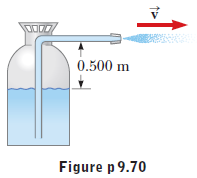# Problem: Water is forced out of a fire extinguisher by air pressure, as shown in the figure below. What gauge air pressure in the tank (above atmospheric pressure) is required for the water to have a jet speed of 30.0 m/s when the water level in the tank is 0.500 m below the nozzle?

###### FREE Expert Solution
96% (357 ratings)
###### Problem Details

Water is forced out of a fire extinguisher by air pressure, as shown in the figure below. What gauge air pressure in the tank (above atmospheric pressure) is required for the water to have a jet speed of 30.0 m/s when the water level in the tank is 0.500 m below the nozzle?What scientific concept do you need to know in order to solve this problem?

Our tutors have indicated that to solve this problem you will need to apply the Bernoulli's Equation concept. If you need more Bernoulli's Equation practice, you can also practice Bernoulli's Equation practice problems.

How long does this problem take to solve?

Our expert Physics tutor, Julia took 3 minutes and 41 seconds to solve this problem. You can follow their steps in the video explanation above.

What professor is this problem relevant for?

Based on our data, we think this problem is relevant for Professor Blng's class at EMORY.

What textbook is this problem found in?

Our data indicates that this problem or a close variation was asked in College Physics - Serway 10th Edition. You can also practice College Physics - Serway 10th Edition practice problems.